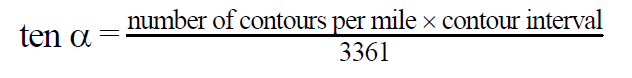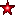# MOCK TEST ON GEOGRAPHICAL TECHNIQUES PYQS JUNE & DEC 2012 NTA UGC NET

MOCK TEST ON GEOGRAPHICAL TECHNIQUES (CARTOGRAPHY & STATS) PYQS JUNE & DEC 2012 NTA UGC NET

Mock Test on GEOGRAPHICAL TECHNIQUES PYQS JUNE 2021 NTA UGC NET, Mock Test on GEOGRAPHICAL TECHNIQUES NTA UGC NET DEC 2020, Mock Test GEOGRAPHICAL TECHNIQUES PYQS UGC NET JUNE 2021 GEOGRAPHICAL TECHNIQUES PYQS DEC 2020, NTA UGC NET PYQS GEOGRAPHY, Mock Test GEOGRAPHICAL TECHNIQUES PYQS UGC NET, GEOGRAPHY PYQS UGC NET, PRACTICAL GEOGRAPHY PYQS NTA UGC NET, CARTOGRAPHY PYQS NTAUGC NET, STATS PYQS NTAUGC NET,

GEOGRAPHICAL TECHNIQUES MOCK TEST 2012

To Attempt this Mock test, Click on the Below Start Button

0%
6
Created on Bynetset corner

2012 CARTOGRAPHY AND STATS PYQS NTA UGC NET

• This Test Contains 25 Questions
• Total Questions is 25
• All Questions are Compulsory
• No Negative Marking
• Specific Time given for this quiz
• Quiz / Test will start after clicking on Start Button
• After Completing Quiz, can see result in Percentages (%)
• Note If you found anything  wrong then please WhatsApp us -8436520537
• Attempt Carefully, so lets start, best wishes

1 / 25

Q.1. Which one of the following is most appropriate for showing frequency distribution? (UGC NET JUNE 2012)

(1) Bar graph

(2) Histogram

(3) Pie diagram

(4) Line graph

2 / 25

Q.2. Which one of the following is commonly used for computing the average value of river discharge? (UGC NET JUNE 2012)

(1) Arithmetic mean

(2) Geometric mean

(3) Harmonic mean

(4) Weighted mean

3 / 25

Q.3. Which one of the following Rn values shows the random pattern of point distribution? (UGC NET JUNE 2012)

(1) 0

(2) 1.0

(3) 1.35

(4) 2.14

4 / 25

Q.4. Which one of the following sampling techniques is most suitable for selection of representative villages of an area with diversified geographical features? (UGC NET JUNE 2012)

(1) Systematic

(2) Stratified - random

(3) Random

(4) Clustered

5 / 25

Q.5. In which one of the following methods interpolation is used? (UGC NET JUNE 2012)

(1) Choroschematic

(2) Chorochromatic

(3) Isopleth

(4) Choropleth

6 / 25

Q.6. The heights of individual points on topographical maps are indicated by: (UGC NET JUNE 2012)

(A) Spot height only

(B) Benchmark only

(C) Triangulation point only

(D) All of the above

7 / 25

Q.7. If point A is at 230 m and point B at 570 m elevations from mean sea level with a horizontal equivalent of 2.0 km, which one of the following gradients is correct between these two points? (UGC NET JUNE 2012)

(A) 0.7 percent

(B) 7.0 percent

(C) 17.0 percent

(D) 27.0 percent

8 / 25

Q.8. Which one of the following techniques is not suitable for showing urban population on the map? (UGC NET JUNE 2012)

(A) Circles

(B) Divided circles

(C) Spheres

(D) Hachures

9 / 25

Q.9. Which one of the following statements is not correct? (UGC NET JUNE 2012)

(A) Contour maps are isopleths maps.

(B) Isopleths are lines of equal value.

(C) Isopleth technique always takes administrative boundaries into account.

(D) Isopleth technique is suitable for continuous data.

10 / 25

Q.10. Consider the following statements and select the correct answer using the codes given below: (UGC NET JUNE 2012)

(1) Deserts, marshes and hilly tracts are known as the negative areas of population.

(2) Dot method is the best technique to show the distribution of population.

(3) Dot method can be used to show age and sex composition.

(4) The dot map cannot be transferred into isopleth.

Codes:

(A) only 1 and 3 are correct.

(B) 1, 2 and 3 are correct.

(C) 1, 2 and 4 are correct.

(D) 1, 2, 3 and 4 are correct.

11 / 25

Q.11. Which of the following is most suitable to show the unequality in the distribution? (UGC NET JUNE 2012)

(A) Lorenz curve

(B) Bar graph

(C) Circle diagram

(D) Isopleth

12 / 25

Q.12. Which one of the following is the correct geometric mean of the data set: 0, 50, 100, 100, 150, 250, 450, 500? (UGC NET JUNE 2012)

(A) 125

(B) 100

(C) 200

(D) 0

13 / 25

Q.13. Which one of the following indicates ‘Random’ distribution of settlements? (UGC NET DEC 2012)

(A) 0.49

(B) 1.00

(C) 1.59

(D) 2.15

14 / 25

Q.14. Which one of the following methods is suitable to represent the proportions of different land uses in a region? (UGC NET DEC 2012)

(A) Line graph

(B) Pie diagram

(C) Hyther graph

(D) Triangular diagram

15 / 25

Q.15. The dot method of distribution refers to (UGC NET DEC 2012)

(A) Iconic Model

(B) Analogue Model

(C) Symbolic Model

(D) Graphical Model

16 / 25

Q.16. Which one of the following is a relative measure of dispersion? (UGC NET DEC 2012)

(A) Standard deviation

(B) Quartile deviation

(C) Coefficient of variation

(D) Range

17 / 25

Q.17. If a map is drawn on RF 1/5500, which one of the following representative fractions will be correct if the map is reduced to half? (UGC NET DEC 2012)

(A) 1/2750

(B) 1/11,000

(C) 1/5,000

(D) 1/33,000

18 / 25

Q.18. Who calculated the slope based on the following formula (UGC NET DEC 2012)(A) Wentworth

(B) Raiz

(C) Henry

(D) Davis

19 / 25

Q.19. Consider the following statements and select the correct answer using codes given below: (UGC NET DEC 2012)

(1) In a Representative Fraction (R.F.), the numerator is always one.

(2) In an R.F. Scale, the unit of denominator is always as of the unit of numerator.

(3) R.F. is not suitable technique of showing scale on a map.

(4) The statement scale, e.g. 1 cm to 1 km is suitable scale on a map.

Codes:

(A) 1, 2 and 3 is correct

(B) 1, 3 and 4 is correct

(C) 2, 3 and 4 is correct

(D) 1, 2 and 4 is correct

20 / 25

Q.20. If Representative Fraction (RF) of a map is 1/5000 which is reduced three times, which of the following RF is correct for reduced map? (UGC NET DEC 2012)

(A) 1/2,500

(B) 1/1,000

(C) 1/15,000

(D) 1/25,000

21 / 25

Q.21. Which one of the following statement is correct in contest of Sten-de-Geer’s method used for showing population distribution? (UGC NET DEC 2012)

(A) Rural population is shown by sphere method

(B) Urban population is shown by dot method

(C) Scale of Cube-roots are used for showing the spheres

(D) Spheres occupy bigger space on map.

22 / 25

Q.22. Which one of the following measurements is used for inequality distribution of farmers’ income subject to their landholding size of an area? (UGC NET DEC 2012)

(A) Standard Deviation

(B) Coefficient of Variation

(C) Gini Index

(D) Correlation coefficient

23 / 25

Q.23. Consider the following statements and select the correct answer using the codes given below: (UGC NET DEC 2012)

(a) Random sampling is called as probability sampling.

(b) Random sampling is done with help of random table.

(c) Landscape elements are generally scattered at random.

Codes:

(A) (a) and (b) are correct.

(B) (b) and (c) are correct.

(C) (a) and (c) are correct.

(D) (a), (b) and (c) are correct.

24 / 25

Q.24. Match List – I with List – II and select the correct answer from the codes given below: (UGC NET DEC 2012)

List – I (Cartographic method)

(a) Chorochromatic

(b) Choroschematic

(c) Isopleth

(d) Choropleth

List – II (Map)

(i) Contour

(iii) Use of letters like R = Rice, W = wheat

(iv) Density of population

Codes:

 a b c d (A) ii iii iv i (B) ii iii i iv (C) iii ii iv i (D) iii ii i iv

25 / 25

Q.25. According to Nearest Neighbour Index, what would be the maximum value for the perfect uniform settlement distribution? (UGC NET DEC 2012)

(A) 0.00

(B) 2.15

(C) 1.55

(D) 2.89

The average score is 63%

0%

## UNIT WISE GEOGRAPHY PYQS MOCK TESTClick Here to Attempt Unit Wise PYQS Mock Test

## MODEL / PRACTICE TEST GEOGRAPHY PAPER 2Click Here to Attempt Model Test

## UNIT WISE GEOGRAPHY MCQS MOCK TESTClick Here to Attempt Unit Wise Mock Test

SHIFT 1 & SHIFT 2 PAPERS DEC 2020 JUNE 2021

(1) DEC 2020 JUNE 2021 SHIFT-1 PAPER 2 GEOGRAPHY NTA UGC NET

(2) DEC 2020 JUNE 2021 SHIFT-2 PAPER 2 GEOGRAPHY NTA UGC NET

Syllabus:

Unit IX:

Geographical Techniques

Sources of Geographic Information and Data (spatial and non-spatial), Types of Maps, Techniques of Map Making (Choropleth, Isarithmic, Dasymetric, Chorochromatic, Flow Maps) Data Representation on Maps (Pie diagrams, Bar diagrams and Line Graph, GIS Database (raster and vector data formats and attribute data formats). Functions of GIS (conversion, editing and analysis), Digital Elevation Model (DEM), Georeferencing (coordinate system and map projections and Datum), GIS Applications ( thematic cartography, spatial decision support system), Basics of Remote Sensing (Electromagnetic Spectrum, Sensors and Platforms, Resolution and Types, Elements of Air Photo and Satellite Image Interpretation and Photogrammetry), Types of Aerial Photographs, Digital Image Processing: Developments in Remote Sensing Technology and Big Data Sharing and its applications in Natural Resources Management in India, GPS Components (space, ground control and receiver segments) and Applications, Applications of Measures of Central Tendency, Dispersion and Inequalities, Sampling, Sampling Procedure and Hypothesis Testing (chi square test, t test, ANOVA), Time Series Analysis, Correlation and Regression Analysis, Measurement of Indices, Making

Indicators Scale Free, Computation of Composite Index, Principal Component Analysis and Cluster Analysis, Morphometric Analysis: Ordering of Streams, Bifurcation Ratio, Drainage Density and Drainage Frequency, Basin Circularity Ratio and Form Factor, Profiles, Slope Analysis, Clinographic Curve, Hypsographic Curve and Altimetric Frequency Graph.

error: Content is protected !!